Benedict, Webb and Rubin equation of state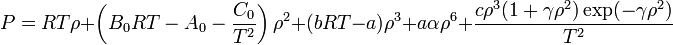$P= RT\rho + \left( B_0 RT - A_0 - \frac{C_0}{T^2} \right)\rho^2 + (bRT -a)\rho^3 + a \alpha \rho^6 + \frac{c\rho^3 ( 1 + \gamma \rho^2) \exp (-\gamma \rho^2)}{T^2}$
where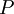$P$ is the pressure,$T$ is the temperature,$\rho$ is the density, and$R$ is the molar gas constant.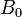$B_0$,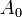$A_0$,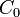$C_0$,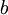$b$,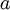$a$,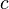$c$,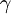$\gamma$ and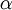$\alpha$ are adjustable parameters.